Download Presentation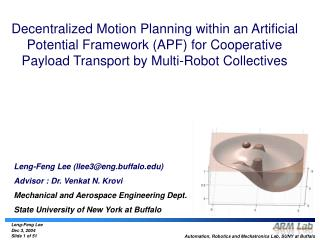Leng-Feng Lee (llee3@eng.buffalo) Advisor : Dr. Venkat N. Krovi Mechanical and Aerospace Engineering Dept. State Univers

# Leng-Feng Lee (llee3@eng.buffalo) Advisor : Dr. Venkat N. Krovi Mechanical and Aerospace Engineering Dept. State Univers - PowerPoint PPT Presentation

Decentralized Motion Planning within an Artificial Potential Framework (APF) for Cooperative Payload Transport by Multi-Robot Collectives Leng-Feng Lee (llee3@eng.buffalo.edu) Advisor : Dr. Venkat N. Krovi Mechanical and Aerospace Engineering Dept. State University of New York at BuffaloI am the owner, or an agent authorized to act on behalf of the owner, of the copyrighted work described.
Download Presentation## Leng-Feng Lee (llee3@eng.buffalo) Advisor : Dr. Venkat N. Krovi Mechanical and Aerospace Engineering Dept. State Univers

An Image/Link below is provided (as is) to download presentation

Download Policy: Content on the Website is provided to you AS IS for your information and personal use and may not be sold / licensed / shared on other websites without getting consent from its author.While downloading, if for some reason you are not able to download a presentation, the publisher may have deleted the file from their server.

- - - - - - - - - - - - - - - - - - - - - - - - - - E N D - - - - - - - - - - - - - - - - - - - - - - - - - -
Presentation Transcript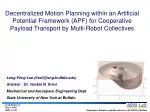Decentralized Motion Planning within an Artificial Potential Framework (APF) for Cooperative Payload Transport by Multi-Robot Collectives

Leng-Feng Lee (llee3@eng.buffalo.edu)

Advisor : Dr. Venkat N. Krovi

Mechanical and Aerospace Engineering Dept.

State University of New York at Buffalo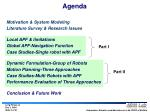Agenda
• Motivation & System Modeling
• Literature Survey & Research Issues
• Local APF & limitations
• Global APF-Navigation Function
• Case Studies-Single robot with APF

Part I

• Dynamic Formulation-Group of Robots
• Motion Planning-Three Approaches
• Case Studies-Multi Robots with APF
• Performance Evaluation of Three Approaches

Part II

• Conclusion & Future Work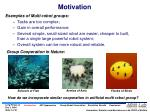Motivation
• Examples of Multi-robot groups:
• Tasks are too complex;
• Gain in overall performance;
• Several simple, small-sized robot are easier, cheaper to built, than a single large powerful robot system;
• Overall system can be more robust and reliable.
• Group Cooperation in Nature:

Armies of Ants

Schools of Fish

Flocks of Birds

• How do we incorporate similar cooperation in artificial multi robot group?

Introduction APF Approaches Group Robot Formulation Simulation Results Conclusion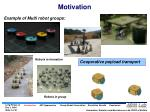Motivation
• Example of Multi robot groups:

Robots in formation

• Cooperative payload transport

Robots in group

Introduction APF Approaches Group Robot Formulation Simulation Results Conclusion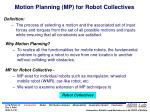Motion Planning (MP) for Robot Collectives
• Definition:
• The process of selecting a motion and the associated set of input forces and torques from the set of all possible motions and inputs while ensuring that all constraints are satisfied.
• Why Motion Planning?
• To realize all the functionalities for mobile robots, the fundamental problem is getting a robot to move from one location to another without colliding with obstacles.
• MP for Robot Collective -
• MP exist for individual robots such as manipulator, wheeled mobile robot (WMR), car-like robot, etc.
• We want to examine extension of MP techniques to
• Robot Collectives

Introduction Model Distribution Analysis Manipulability Cooperative System Conclusion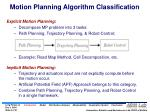Motion Planning Algorithm Classification
• Explicit Motion Planning:
• Decompose MP problem into 3 tasks:
• Path Planning, Trajectory Planning, & Robot Control;
• Example: Road Map Method, Cell Decomposition, etc.
• Implicit Motion Planning:
• Trajectory and actuators input are not explicitly compute before the motion occur.
• Artificial Potential Field (APF) Approach belongs to this category.
• Combine Path Planning, Trajectory Planning, and Robot Control in a single framework.

Introduction Model Distribution Analysis Manipulability Cooperative System Conclusion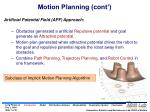Motion Planning (cont’)
• Artificial Potential Field (APF) Approach:
• Obstacles generated a artificial Repulsive potential and goal generate an Attractive potential.
• Motion plan generated when attractive potential drives the robot to the goal and repulsive potential repels the robot away from obstacles.
• Combine Path Planning, Trajectory Planning, and Robot Control in one framework.

Subclass of Implicit Motion Planning Algorithm

Introduction Model Distribution Analysis Manipulability Cooperative System ConclusionResearch Issues
• Extending APF approach for Multi-robot collectives.
• Ensuring tight formations required for Cooperative Payload Transport application.
• Specific Research Questions:
• Which type of potential function is more suitable for MP for multi robot groups?
• How can we use the APF framework to help maintain formation? and
• How this framework be extended to realize the tight formation requirement for cooperative payload transport?

Introduction Model Distribution Analysis Manipulability Cooperative System Conclusion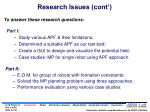Research Issues (cont’)
• To answer these research questions:
• Part I:
• Study various APF & their limitations;
• Determined a suitable APF as our test bed;
• Create a GUI to design and visualize the potential field;
• Case studies: MP for single robot using APF approach.
• Part II:
• E.O.M. for group of robots with formation constraints;
• Solved the MP planning problem using three approaches;
• Performance evaluation using various case studies.

Introduction Model Distribution Analysis Manipulability Cooperative System Conclusion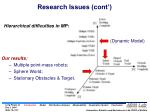Research Issues (cont’)
• Hierarchical difficulties in MP:

(Dynamic Model)

• Our results:
• Multiple point-mass robots;
• Sphere World;
• Stationary Obstacles & Target.

Introduction Model Distribution Analysis Manipulability Cooperative System ConclusionSystem Modeling
• Individual level system models include:
• Point Mass Robot;
• Differentially Driven Nonholonomic Wheel Mobile Robot (NH-WMR).

Introduction Model Distribution Analysis Manipulability Cooperative System ConclusionSystem Modeling (cont’)
• Group level system model is formed using:
• Point Mass Robot;
• Differentially Driven Nonholonomic Wheel Mobile Robot.

Introduction Model Distribution Analysis Manipulability Cooperative System ConclusionPART I: Artificial Potential Approach
• Examine:
• Variants of APF & their limitations;
• Navigation function ;
• Single module formulations;
• Simulation studies.Local APF -background
• Artificial Potential Field Approach
• Proposed by Khatib in early 80’s.
• FIRAS Function. [Khatib, 1986]
• Later, various kind of Potential Functions were proposed:
• GPF Function. [Krogh, 1984]
• Harmonic Potential Function. [Kim, 1991]
• Superquadric Potential Function. [Khosla, 1988]
• Navigation Function.[Koditschek, 1988]
• Ge New Potential. [Ge, 2000]

Introduction APF Approaches Group Robot Formulation Simulation Results ConclusionLocal APF Approach-Formulation
• Idea:
• Goal generate an attractive potential well;
• Obstacle generate repulsive potential hill;
• Superimpose these two type of potentials give us the total potential of the workspace.

Where:

denote the total artificial potential field;

denote the attractive potential field; and

is the repulsive potential field.

is the position of the robot.

Introduction APF Approaches Group Robot Formulation Simulation Results ConclusionLocal APF -Attractive potential
• Characteristics:
• Affect every point on the configuration space;
• Minimum at the goal.
• The gradient must be continuous.

Introduction APF Approaches Group Robot Formulation Simulation Results ConclusionLocal APF -Attractive potential
• Example 1:

Where:

= Positive scaling factor

= Euclidean distance between the robot and the target

= Position of the target.

= Position of the robot.

is commonly used.

Introduction APF Approaches Group Robot Formulation Simulation Results ConclusionLocal APF -Attractive potential
• Example 2:

Where:

= Positive scaling factor

For distance smaller than s, conical well.

For distance larger than s, constant attractive force.

Introduction APF Approaches Group Robot Formulation Simulation Results ConclusionLocal APF -Repulsive potential
• Characteristics:
• The potential should have spherical symmetry for large distance;
• The potential contours near the surface should follow the surface contour;
• The potential of an obstacle should have a limited range of influence;
• The potential and the gradient of the potential must be continuous.

Introduction APF Approaches Group Robot Formulation Simulation Results ConclusionLocal APF -Repulsive potential
• Example 1 - FIRAS Function:

Where:

= Positive scaling factor

= the shortest Euclidean distance

between the robot from the obstacle surface

Introduction APF Approaches Group Robot Formulation Simulation Results ConclusionLocal APF -Repulsive potential
• Example 2 - Superquadric Potential Function:
• Approach Potential;
• Avoidance Potential.
• Avoid creation of local minima result from flat surface by creating a symmetry contour around the obstacle.

Introduction APF Approaches Group Robot Formulation Simulation Results ConclusionLocal APF -Repulsive potential
• Example 3 - Harmonic Potential Function:

Attractive Potential

Repulsive Potential

• Superimpose of another harmonic potential is also a harmonic potential.
• More complicated shape can be modeled using ‘panel method’.

Detail

Introduction APF Approaches Group Robot Formulation Simulation Results ConclusionLocal APF -Repulsive potential
• Example 4 - Ge New Function:

Where:

= Minimal Euclidean distance

from robot to the target.

• Modified from FIRAS function to solve the ‘Goal NonReachable for Obstacle Nearby’ -GNRON problem.
• Ensures that the total potential will reach its global minimum, if and only if the robot reaches the target where

Introduction APF Approaches Group Robot Formulation Simulation Results ConclusionLocal APF -Repulsive potential
• Potential Function with Velocities Information:
• Some potential function include the velocities information of the robots, obstacles and target.
• Example: Ge & Cui Potential [Dynamic obstacle & Target].
• Provide a APF for dynamic workspace.
• Example: GPF Function. [Dynamic obstacles only].
• Can be used with our formulation for group of robots for motion planning in dynamic workspace.

Introduction APF Approaches Group Robot Formulation Simulation Results ConclusionLocal APF –Total Potential
• Total Potential of Workspace:
• Superimpose different repulsive potential from obstacles and different attractive potential from the goal, we get the total potential for the workspace.
• At any point of the workspace, the robot will reach the target byfollowing the negative gradient flow of the total potential.

Introduction APF Approaches Group Robot Formulation Simulation Results ConclusionLocal APF –Total Potential
• Example: FIRAS Function

Rectangular Obstacle:

Circular Obstacle:

2 unit in height, 1 unit in width.

Target :

More

Introduction APF Approaches Group Robot Formulation Simulation Results ConclusionLocal APF –Limitations
• Local Minimum - result from single obstacle

3D View

Introduction APF Approaches Group Robot Formulation Simulation Results ConclusionLocal APF –Limitations
• Local Minimum - result from multiple obstacles

Introduction APF Approaches Group Robot Formulation Simulation Results ConclusionLocal APF –Limitations
• Limitation - Target close to obstacle:

Introduction APF Approaches Group Robot Formulation Simulation Results ConclusionLocal APF -Limitations
• Some other limitations include:
• No passage between closely spaced obstacle.
• Non optimal path.
• Implementation related limitations.
• Oscillation in the presence of obstacle;
• Oscillation in narrow passages;
• Infinite torque is not possible.

Introduction APF Approaches Group Robot Formulation Simulation Results ConclusionGlobal APF – Navigation Function

[ Proposed by: Rimon & Koditschek]

• Properties:
• Guarantee to provide a global minimum at target.
• Bounded maximum potential.

Let

be a robot free configuration space, and let

be a goal point in the interior of

, A map

is a Navigation Function if it is:

.

function.

, that is, at least a

1. Smooth on

.

2. Polar at

,i.e., has a unique minimum at

on the path-connected

component of

containing

, i.e., uniformly maximal on the boundary of

4. A Morse Function

Introduction APF Approaches Group Robot Formulation Simulation Results ConclusionFeature: Tunable by a single parameter :

• Navigation Function of a sphere world :

Where:

Detail

is the implicit form of bounding sphere.

is the implicit form of obstacle geometric Eq.

Number of obstacles

Introduction APF Approaches Group Robot Formulation Simulation Results Conclusion• Example - Navigation Function of a sphere world :

Where:

Introduction APF Approaches Group Robot Formulation Simulation Results Conclusion• At low value of , local minima may exist:

Introduction APF Approaches Group Robot Formulation Simulation Results ConclusionNavigation Function – MATLAB GUI
• A GUI to properly select a value:

Introduction APF Approaches Group Robot Formulation Simulation Results ConclusionAPF Approach – Formulation & Simulation
• Idea:
• We want the robot to follow the negative gradient flow of the workspace potential field;
• Analogy to a ball rolling down to the lowest point in a given potential.
• Thus the gradient information will serve as the input to the robot system.

Introduction APF Approaches Group Robot Formulation Simulation Results ConclusionAPF Approach – Formulation
• Formulation – Single point-mass robot:

Kinematic Model:

Dynamic Model:

is a positive diagonal scaling matrix

is the gradient of the potential field

is dissipative term added to stabilize the system

Introduction APF Approaches Group Robot Formulation Simulation Results ConclusionAPF Approach – Formulation
• Formulation – Nonholonomic Wheeled Mobile Robot (NH-WMR):

Kinematic Model:

is the projected gradient onto the direction of forward velocity.

is the proportional to the angular error between the gradient and robot direction.

the desired x-direction velocity.

desired y-direction velocity.

Introduction APF Approaches Group Robot Formulation Simulation Results ConclusionAPF Approach – Formulation
• Formulation – Group robot without formation constraints:

Generalize position:

-number of point-mass robot

Kinematic Model:

Dyanamic Model:

Introduction APF Approaches Group Robot Formulation Simulation Results ConclusionAPF Approach – Simulations
• Simulation 1 – Single robot with single obstacle:

Detail

Introduction APF Approaches Group Robot Formulation Simulation Results ConclusionAPF Approach – Simulations
• Simulation 2 – Single robot with two obstacles:

Detail

Introduction APF Approaches Group Robot Formulation Simulation Results ConclusionAPF Approach – Simulations
• Simulation 3 – Single NH-WMR with four obstacles:

Detail

More

Introduction APF Approaches Group Robot Formulation Simulation Results ConclusionAPF Approach – Simulations
• Simulation 4 – Group robots without formation constraint:

Detail

Introduction APF Approaches Group Robot Formulation Simulation Results ConclusionAPF Approach – Simulations
• Simulation 5 – Group robots without formation constraint:

Detail

Introduction APF Approaches Group Robot Formulation Simulation Results ConclusionPART II: Group Robots Dynamic Formulation
• Include:
• Dynamic Formulation for Group of Robots with Formation;
• Solved the E.O.M using three Methods;
• Simulation Studies;
• Performance evaluation of each Methods.Group Robots Dynamic Formulation
• Approaches for formation maintenance:
• Leader-follower [Desai et. al., 2001]
• Virtual structures [Lewis and Tan, 1997]
• Virtual leaders [Leonard and Fiorelli, 2001], [Lawton, Beard et al., 2003]
• Our Approaches:
• View as a constrained mechanical system.
• Formation constraints – holonomic constraints added to a unconstrained dynamic system.
• Motion planning now can be treated as a forward dynamic simulation of a constrained mechanical system.

Introduction APF Approaches Group Robot Formulation Simulation Results ConclusionGroup Robots Dynamic Formulation
• The dynamic of group of robot can be formulated using Lagrange Equation by:

(1)

is the n-dimensional vector of generalized coordinates

is the n-dimensional vector of generalized velocities

is the n-dimensional vector of generalized velocities

is the n-dimensional vector of external forces

is the vector of input forces, which is

is the Jacobian matrix

Introduction APF Approaches Group Robot Formulation Simulation Results ConclusionGroup Robots Dynamic Formulation
• The Lagrange Equation can be solved using following three methods:
• Method I: Direct Lagrange Multiplier Elimination Approach.
• Explicitly computing the Lagrange multiplier by a projection into the constrained force space.
• Method II: Penalty Formulation Approach.
• Approximating the Lagrange multiplier using artificial compliance elements such as virtual springs and dampers.
• Method III: Constraints Manifold Projection Based Approach
• By projecting the equations of motion onto the tangent space of the constraint manifold in a variety of ways to obtain constraint-reaction free equations of motions.

Introduction APF Approaches Group Robot Formulation Simulation Results ConclusionGroup Robots Dynamic Formulation
• Method I: Direct Lagrange Multiplier Elimination Approach:
• The direct Lagrange multiplier elimination is a centralized approach where the Lagrange multiplier is explicitly calculated to ensure formation constraints are not violated.

(2)

The resulting Dynamic Equation can be expressed as:

(3)

Detail

Introduction APF Approaches Group Robot Formulation Simulation Results ConclusionGroup Robots Dynamic Formulation
• Method II: Penalty Formulation Approach:
• The holonomic constraints are relaxed and replaced by linear/non-linear spring with dampers.
• Here, the Lagrange multipliers are explicitly approximated as the force of a virtual spring or damper based on the extent of the constraint violation and assumed spring stiffness and damping constant.

This can be expressed as:

Resulting Dynamic Equation:

(4)

Introduction APF Approaches Group Robot Formulation Simulation Results ConclusionGroup Robots Dynamic Formulation
• Method III: Constraints Manifold Projection Based Approach:
• In this approach, the dynamic equation with constraint-reactions is projected into the tangent space (feasible motion subspace) to obtain the constraint free projected dynamics equations.

Is the independent velocities.

Thus, the resulting Dynamic Equation become:

(5)

Detail

Introduction APF Approaches Group Robot Formulation Simulation Results ConclusionGroup Robots Dynamic Formulation
• Baumgarte Stabilization:
• To prevent numerical drift in the simulation, we adopted Baumgarte stabilization method.
• Baumgarte stabilization method involves the creation of an artificial first or second order dynamical system which has the algebraic position-level constraint as its attractive equilibrium configuration.

For example, the holonomic constraint of Eq.(1) is replaced with:

Where the solution of the above equation is :

Introduction APF Approaches Group Robot Formulation Simulation Results ConclusionCase Study - Formulation:
• Three point-mass robots forming a triangular shape:

The governing Equation can be written as:

where:

We will use this model to perform various case studies.

Introduction APF Approaches Group Robot Formulation Simulation Results ConclusionSimulation & Results
• Performance Evaluation – Formation Error:

Formation Error:

is the total formation error;

is the actual Euclidean distance between robot

and robot

and robot

is the desired Euclidean distance between robot

Introduction APF Approaches Group Robot Formulation Simulation Results ConclusionSimulations & Results
• Case Study 1 – Three robots in formation, without obstacle:

Method I:

Introduction APF Approaches Group Robot Formulation Simulation Results ConclusionSimulations & Results
• Case Study 1 – Three robots in formation, without obstacle:

Method II:

Decentralized Formulation:

Introduction APF Approaches Group Robot Formulation Simulation Results ConclusionSimulation & Results
• Case Study 1 – Three robots in formation, without obstacle:

Method III:

Partial Decentralized Formulation:

Introduction APF Approaches Group Robot Formulation Simulation Results ConclusionSimulation & Results
• Case Study 1 – Formation Error from three methods:

Method I

Method II

Method III

Introduction APF Approaches Group Robot Formulation Simulation Results ConclusionSimulation & Results
• Case Study 1 – Formation Error & Effect of Ks

Method II:

Introduction APF Approaches Group Robot Formulation Simulation Results ConclusionSimulation & Results
• Case Study 1 – Formation Error & Effect of

Method II:

Introduction APF Approaches Group Robot Formulation Simulation Results ConclusionSimulation & Results
• Case Study 2 – Three robots in Formation, one obstacle

Introduction APF Approaches Group Robot Formulation Simulation Results ConclusionSimulations & Results
• Case Study 2 – Three robots in formation, one obstacle:

Method I

Method III

Method II

Introduction APF Approaches Group Robot Formulation Simulation Results ConclusionSimulation & Results
• Case Study 2 – Formation Error from three methods:

Method I

Method II

Method III

Introduction APF Approaches Group Robot Formulation Simulation Results ConclusionSimulation & Results
• Case Study 2 – Formation Error & Effect of Ks &

Method II

Method III

Introduction APF Approaches Group Robot Formulation Simulation Results ConclusionSimulation & Results
• Case Study 2 – Three robots in Formation with Expansion.

Each sides change from 2 units to 4 units in 4 seconds:

Introduction APF Approaches Group Robot Formulation Simulation Results ConclusionSimulation & Results
• Case Study 3 – Three robots in Formation with Expansion.

Method I

Method III

Method II

Introduction APF Approaches Group Robot Formulation Simulation Results ConclusionSimulation & Results
• Case Study 3 – Formation Error & Effect of Ks &

Method II

Method III

Introduction APF Approaches Group Robot Formulation Simulation Results ConclusionSimulation & Results
• Case Study 4 – Three robots in Formation with Shape Change.

Constraint between robot A & B change from 2 units to 4 units in 4 seconds:

Note: Method I cannot perform this task because when three robots in a straight line, the inverse of the Jacobian matrix become singular.

Introduction APF Approaches Group Robot Formulation Simulation Results ConclusionSimulation & Results
• Case Study 4 – Three robots in Formation with Shape Change.

Method II

Method III

Introduction APF Approaches Group Robot Formulation Simulation Results ConclusionSimulation & Results
• Case Study 4 – Formation Error & Effect of Ks &

Method II:

Method III:

Introduction APF Approaches Group Robot Formulation Simulation Results ConclusionConclusion
• General Characteristics – Formation Accuracy

The average total formation error for each method :

Introduction APF Approaches Group Robot Formulation Simulation Results ConclusionConclusion
• General Characteristics – Computational Time

The average total Computational Time (sec) for each method :

Introduction APF Approaches Group Robot Formulation Simulation Results ConclusionConclusion
• General Characteristics – Decentralize formulation capability

The decentralize formulation capability for each method :

Centralized Decentralized

Introduction APF Approaches Group Robot Formulation Simulation Results ConclusionConclusion
• General Characteristics – Formation relatedconcerns:
• The Jacobian matrix in Method I and Method III can become singular in some specific position.
• Method II has no such limitations.

In Summary:

Introduction APF Approaches Group Robot Formulation Simulation Results ConclusionConclusion
• Evaluation of various potential functions.
• Development of a GUI to generate navigation function.
• Develop the group motion planning problem as a forward dynamic simulation problem;
• Evaluation of three different method in solving motion planning problem for a group of robots in formation.
• Critical evaluation of the performance by the three approaches.

Introduction APF Approaches Group Robot Formulation Simulation Results ConclusionFuture Work
• Provide a way to avoid Jacobian matrix become singular.
• Incorporate nonholonomic constraints in the formulation.
• Implement a more efficient gradient finding method by utilizing the available information from each robot.
• Implement the algorithm in a decentralized computation manner.

Introduction Model Distribution Analysis Manipulability Cooperative System Conclusion### Thank You!

Acknowledgments:

Dr. V. Krovi, Dr. T. Singh & Dr. J. L. Crassidis